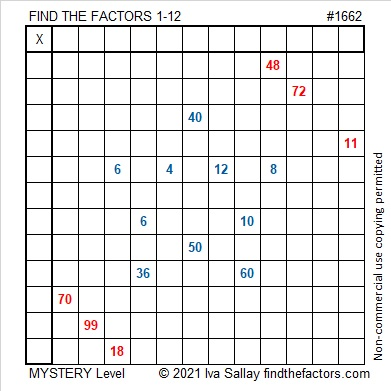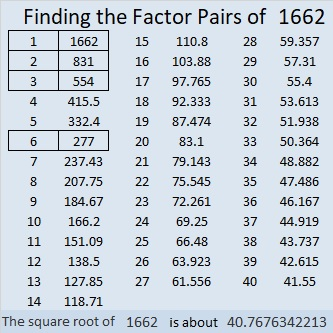### Today’s Puzzle:

Tomorrow is Independence Day in the United States. Happy Independence Day! Wherever you live, you can have a different kind of independence day, and it can happen any day of the year:

Are you dependent on a calculator, Siri, or someone or something else to give you any of the products or divisors in a multiplication table? Solving these Find the Factors puzzles can help you be more familiar with the table and declare your independence from those outside sources! Use logic to help you find its unique solution. Yes, mystery-level puzzles can be tricky, but I’ll give you a hint under the puzzle if you need it.The logic to get started: One column has 40, 50 and another column has 10, 60. There are only two numbers that can go at the top of either one of those columns: 5 and 10. We don’t know which column gets which number, however. But it is still enough to tell us that the other 5 and 10 must go in the first column with the 10 being a factor of 70 and the 5 being a factor of 50.

### Factors of 1662:

1662 is even, so it is divisible by 2.
1 + 2 = 3, so 1662 is divisible by 3. (Why wasn’t it necessary to include the 6’s in that calculation?)
Since 1662 is divisible by both 2 and 3, it is divisible by 6, too.

• 1662 is a composite number.
• Prime factorization: 1662 = 2 × 3 × 277.
• 1662 has no exponents greater than 1 in its prime factorization, so √1662 cannot be simplified.
• The exponents in the prime factorization are 1, 1, and 1. Adding one to each exponent and multiplying we get (1 + 1)(1 + 1)(1 + 1) = 2 × 2 × 2 = 8. Therefore 1662 has exactly 8 factors.
• The factors of 1662 are outlined with their factor pair partners in the graphic below.### More About the Number 1662:

1662 is the hypotenuse of a Pythagorean triple:
690-1512-1662, which is 6 times (115-252-277).
Did you notice all the repeating digits in that triple?